## Example Questions

### Example Question #1 : How To Find The Equation Of A Curve

Solve the equation for x and y.

x² + y = 31

x + y = 11

x = 13, 7

y = 8, –6

x = 6, 15

y = 5, –4

x = 8, –6

y = 13, 7

x = 5, –4

y = 6, 15

x = 5, –4

y = 6, 15

Explanation:

Solving the equation follows the same system as the first problem. However since x is squared in this problem we will have two possible solutions for each unknown. Again substitute y=11-x and solve from there. Hence, x2+11-x=31. So x2-x=20. 5 squared is 25, minus 5 is 20. Now we know 5 is one of our solutions. Then we must solve for the second solution which is -4. -4 squared is 16 and 16 –(-4) is 20. The last step is to solve for y for the two possible solutions of x. We get 15 and 6. The graph below illustrates to solutions.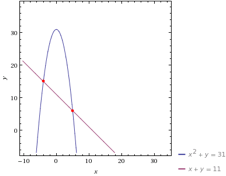### Example Question #1 : X And Y Intercept

Solve the equation for x and y.

x² – y = 96

x + y = 14

x = 5, –14

y = 15, 8

x = 25, 4

y = 10, –11

x = 15, 8

y = 5, –14

x = 10, –11

y = 25, 4

x = 10, –11

y = 25, 4

Explanation:

This problem is very similar to number 2. Derive y=14-x and solve from there. The graph below illustrates the solution.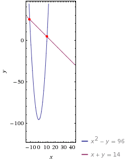### Example Question #3 : How To Find The Equation Of A Curve

Solve the equation for x and y.

5x² + y = 20

x² + 2y = 10

No solution

x = √10/3, –√10/3

y = 10/3

x = 14, 5

= 4, 6

x = √4/5, 7

= √3/10, 4

x = √10/3, –√10/3

y = 10/3

Explanation:

The problem involves the same method used for the rest of the practice set. However since the x is squared we will have multiple solutions. Solve this one in the same way as number 2. However be careful to notice that the y value is the same for both x values. The graph below illustrates the solution.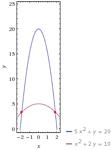### Example Question #4 : How To Find The Equation Of A Curve

Solve the equation for x and y.

x² + y = 60

x – y = 50

x = 40, 61

y = 11, –10

x = 11, –10

y = 40, 61

x = 10, –11

y = –40, –61

x = –40, –61

y = 10, –11

x = 10, –11

y = –40, –61

Explanation:

This is a system of equations problem with an x squared, to be solved just like the rest of the problem set. Two solutions are required due to the x2. The graph below illustrates those solutions.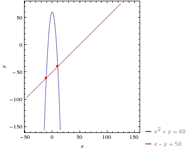### Example Question #5 : How To Find The Equation Of A Curve

A line passes through the points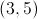and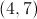. What is the equation for the line?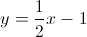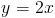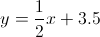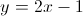Explanation:

First we will calculate the slope as follows: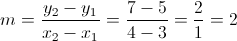And our equation for a line is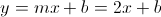Now we need to calculate b. We can pick either of the points given and solve for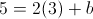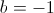Our equation for the line becomes